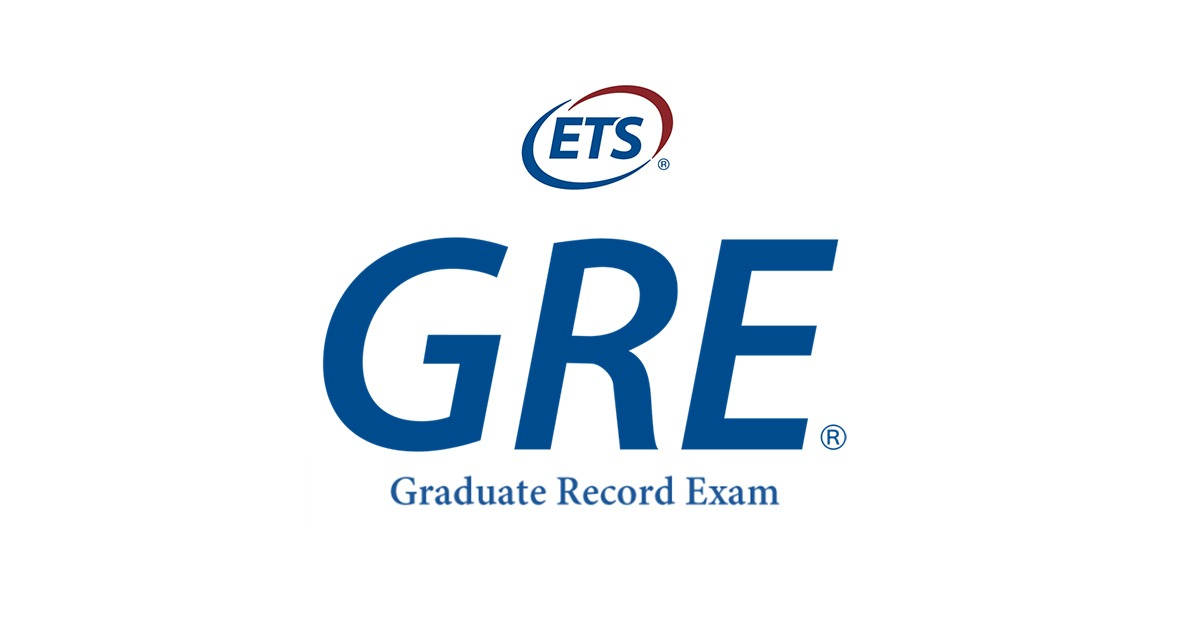info@universityleap.com +91 9899101239, 01149322334

# Find out what GRE Quantitative Reasoning looks like?

> > > Find out what GRE Quantitative Reasoning looks like?When any student looks out opportunities for pursuing a Masters (MBA) Degree abroad, there can be speculations one can have in mind regarding the process. It is at that time that a student learns about certain standardised tests that make the admission process easier. One such test is the GRE (Graduate Record Examination) where the verbal, quantitative and analytical skills of students are taken into account. There are several universities in the USA and many other countries, where the GRE score is considered for admission in their Masters’ programmes.

As the GRE has multiple stages in its completion, it is to be noted that the first stage is the Quantitative one which consists of different parts within itself. In the first part, the students can find a medium-difficulty level of questions. The difficulty of the questions in the next part is based on the performance of the students in the first part. Similar is the scenario when it comes to the verbal section of the GRE.

The questions in the quantitative reasoning (quant section) are based on the following content areas:

Arithmetic: This includes questions from topics such as number line, number sequences, exponents & roots, prime numbers, factorisation, divisibility, types of integers, etc.

Algebra: It’s to do basically with factoring & simplifying algebraic expressions, solving linear & quadratic equations/inequalities, creating equations from word problems, coordinate geometry, intercepts & slopes of lines, etc.

Geometry: Questions range about lines, circles, triangles and their types. Refresh your mind with little reference to quadrilaterals, Pythagoras theorem and other three-dimensional figures.

Data Analysis: The high school mathematics comes to use here, like in the above content areas. Get set ready, to answer questions on mean, median, mode, range, standard deviation along with interpretations of data in the tabular format or graphically.The tentative number of questions in the GRE Quantitative Reasoning Section are 40 that need to be answered in 70 minutes timeline. The section is further divided into two parts of 35 minutes and 20 questions each. There are four types of questions in the Quant section. They are given below:

• Quantitative Comparison Questions
• Multiple Choice Questions (Select one)
• Multiple Choice Questions (Select more than one answer)
• Numeric Entry Questions

For assistance in preparing for your GRE get in touch with University Leap, and get expert career counselling in Delhi.